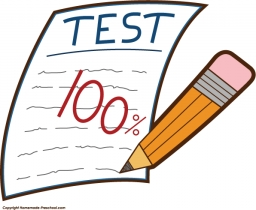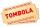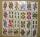# The test

The test contains four questions, and there are five different answers to each of them, of which only one is correct, the others are incorrect. What is the probability that a student who does not know the answer to any question will guess the right answers to all the questions?

Result

p =  0.16 %

#### Solution:

$p=100 \cdot \ (1/5)^{ 4 }=\dfrac{ 4 }{ 25 }=0.16=0.16 \%$Our examples were largely sent or created by pupils and students themselves. Therefore, we would be pleased if you could send us any errors you found, spelling mistakes, or rephasing the example. Thank you!

Leave us a comment of this math problem and its solution (i.e. if it is still somewhat unclear...):

Showing 1 comment:Math student
You make it simple where I can understand thank you so muchTips to related online calculators
Would you like to compute count of combinations?

## Next similar math problems:

1. ClassroomOf the 26 pupils in the classroom, 12 boys and 14 girls, four representatives are picked to the odds of being: a) all the girls b) three girls and one boy c) there will be at least two boysThere are 15 boys and 12 girls at the graduation party. Determine how many four couples can be selected.
3. Three workplacesHow many ways can we divide nine workers into three workplaces if they need four workers in the first workplace, 3 in the second workplace and 2 in the third?
4. Families 2There are 729 families having 6 children each. The probability of a girl is 1/3 and the probability of a boy is 2/3. Find the the number of families having 2 girls and 4 boys.
5. Boys and girlsThere are 11 boys and 18 girls in the classroom. Three pupils will answer. What is the probability that two boys will be among them?
6. Boys and girlsThere are eight boys and nine girls in the class. There were six children on the trip from this class. What is the probability that left a) only boys b) just two boys
7. RaffleThere are 200 draws in the raffle, but only 20 of them win. What is the probability of at least 4 winnings for a group of people who have bought 5 tickets together?
8. STRESSED wordEach letter in STRESSED is printed on identical cards, one letter per card and assembled in random order. Calculate the probability that the cards spell DESSERTS when assembled.
9. Two groupsThe group of 10 girls should be divided into two groups with at least 4 girls in each group. How many ways can this be done?
10. SalamiHow many ways can we choose 5 pcs of salami if we have 6 types of salami for 10 pieces and one type for 4 pieces?How many ways can we thread 4 red, 5 blue, and 6 yellow beads onto a thread?How many words can be created from the word MATEMATIKA by changing the order of the letters, regardless of whether or not the words are meaningful?What is the probability that two dice fall will have the sum 7 or product 12?What is the probability that when choosing 3 carats from seven carats, all 3 reds will be red?From a set of 32 cards we randomly pull out three cards. What is the probability that it will be seven king and ace?I have 4 sweaters two are white, 1 red and 1 green. How many ways can this done?What is the probability that the two throws of the dice: a) Six falls even once b) Six will fall at least once